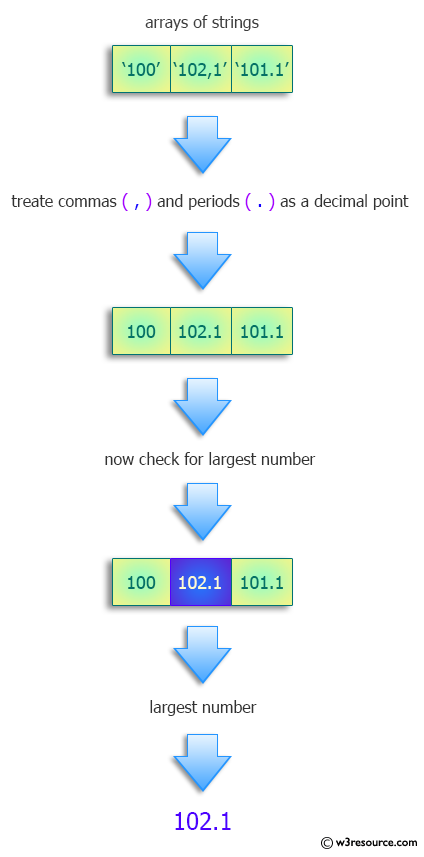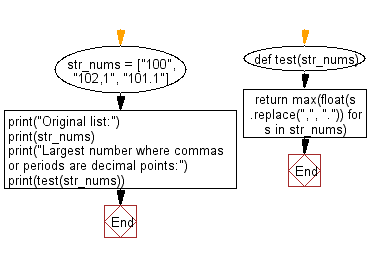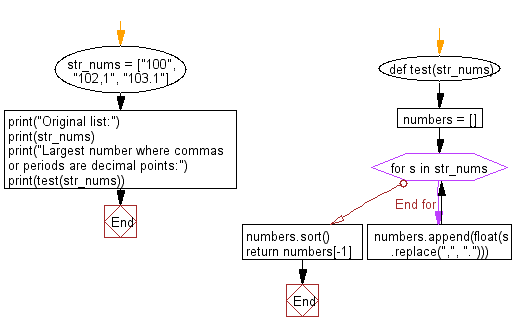﻿ Python: Find the largest number where commas or periods are decimal points - w3resource# Python: Find the largest number where commas or periods are decimal points

## Python Programming Puzzles: Exercise-26 with Solution

Write a Python program to find the largest number where commas or periods are decimal points.

```Input:
['100', '102,1', '101.1']
Output:
102.1
```

Pictorial Presentation:Sample Solution-1:

Python Code:

``````#License: https://bit.ly/3oLErEI

def test(str_nums):
return max(float(s.replace(",", ".")) for s in str_nums)
str_nums = ["100", "102,1", "101.1"]
print("Original list:")
print(str_nums)
print("Largest number where commas or periods are decimal points:")
print(test(str_nums))
``````

Sample Output:

```Original list:
['100', '102,1', '101.1']
Largest number where commas or periods are decimal points:
102.1
```

Flowchart:## Visualize Python code execution:

The following tool visualize what the computer is doing step-by-step as it executes the said program:

Sample Solution-2:

Python Code:

``````#License: https://bit.ly/3oLErEI

def test(str_nums):
numbers = []
for s in str_nums:
numbers.append(float(s.replace(",", ".")))
numbers.sort()
return numbers[-1]

str_nums = ["100", "102,1", "103.1"]
print("Original list:")
print(str_nums)
print("Largest number where commas or periods are decimal points:")
print(test(str_nums))
``````

Sample Output:

```Original list:
['100', '102,1', '103.1']
Largest number where commas or periods are decimal points:
103.1
```

Flowchart:## Visualize Python code execution:

The following tool visualize what the computer is doing step-by-step as it executes the said program:

Python Code Editor :

Have another way to solve this solution? Contribute your code (and comments) through Disqus.

What is the difficulty level of this exercise?

Test your Programming skills with w3resource's quiz.

﻿

## Python: Tips of the Day

Clamps num within the inclusive range specified by the boundary values x and y:

Example:

```def tips_clamp_num(num,x,y):
return max(min(num, max(x, y)), min(x, y))
print(tips_clamp_num(2, 4, 6))
print(tips_clamp_num(1, -1, -6))
```

Output:

```4
-1
```